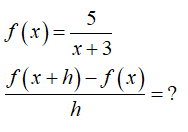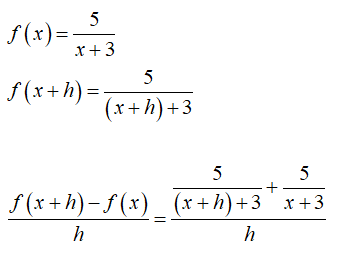# Evaluate and simplify the difference quotient, (f(x+h)-f(x))/h for f(x)=5/(x+3).

Question
3 views

Evaluate and simplify the difference quotient, (f(x+h)-f(x))/h for f(x)=5/(x+3).

check_circle

Step 1

Given:Step 2

Calculation...

### Want to see the full answer?

See Solution

#### Want to see this answer and more?

Solutions are written by subject experts who are available 24/7. Questions are typically answered within 1 hour.*

See Solution
*Response times may vary by subject and question.
Tagged in

### Calculus